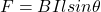## The magnitude of the magnetic force on a current-carrying wire held in a magnetic field depends on the strength of the magnetic

Question

The magnitude of the magnetic force on a current-carrying wire held in a magnetic
field depends on
the strength of the magnetic field
the current in the wire
the length of the wire in the magnetic field
all of the above

in progress 0
7 months 2021-07-13T20:41:30+00:00 1 Answers 4 views 0

All of the above

Explanation:

The magnitude of the magnetic force on a current-carrying wire held in a magnetic is given by the equationWhere B = Strength of the magnetic field

I = The current carried by the wire

l = length of the wire in the magnetic field

θ = Angle between the wire and the magnetic field

Based on the relationship written above, the magnitude of the magnetic force on the current – carrying wire in the magnetic field depends on the strength of the magnetic field (B), length of the wire(l), current in the wire (I).

All the options are correct.